# Interpreting regression coefficients (including interaction coefficients)

A short tutorial on how to interpreting regression coefficients, including interaction coefficients.

Hause Lin true
07-06-2019

Get source code for this RMarkdown script here.

## Consider being a patron and supporting my work?

Donate and become a patron: If you find value in what I do and have learned something from my site, please consider becoming a patron. It takes me many hours to research, learn, and put together tutorials. Your support really matters.

This tutorial provides a step-by-step introduction to interpreting regression coefficients in linear models. I will use the built-in dataset `mtcars`.

General guidelines for interpreting regression coefficients

• intercept coefficient term (b0): the value of the outcome variable when all predictors = 0
• all other non-interaction coefficients: the change in the outcome variable when the predictor increases by 1
• interaction coefficients: the change in a coefficient value when one predictor increases by 1
``````
library(data.table)  # to manipulate dataframes
library(interactions)  # to plot interactions later on
library(ggplot2)``````

Have a look at the `mtcars` dataset.

``````
dt1 <- as.data.table(mtcars)  # convert to datatable
dt1``````
``````
mpg cyl  disp  hp drat    wt  qsec vs am gear carb
1: 21.0   6 160.0 110 3.90 2.620 16.46  0  1    4    4
2: 21.0   6 160.0 110 3.90 2.875 17.02  0  1    4    4
3: 22.8   4 108.0  93 3.85 2.320 18.61  1  1    4    1
4: 21.4   6 258.0 110 3.08 3.215 19.44  1  0    3    1
5: 18.7   8 360.0 175 3.15 3.440 17.02  0  0    3    2
6: 18.1   6 225.0 105 2.76 3.460 20.22  1  0    3    1
7: 14.3   8 360.0 245 3.21 3.570 15.84  0  0    3    4
8: 24.4   4 146.7  62 3.69 3.190 20.00  1  0    4    2
9: 22.8   4 140.8  95 3.92 3.150 22.90  1  0    4    2
10: 19.2   6 167.6 123 3.92 3.440 18.30  1  0    4    4
11: 17.8   6 167.6 123 3.92 3.440 18.90  1  0    4    4
12: 16.4   8 275.8 180 3.07 4.070 17.40  0  0    3    3
13: 17.3   8 275.8 180 3.07 3.730 17.60  0  0    3    3
14: 15.2   8 275.8 180 3.07 3.780 18.00  0  0    3    3
15: 10.4   8 472.0 205 2.93 5.250 17.98  0  0    3    4
16: 10.4   8 460.0 215 3.00 5.424 17.82  0  0    3    4
17: 14.7   8 440.0 230 3.23 5.345 17.42  0  0    3    4
18: 32.4   4  78.7  66 4.08 2.200 19.47  1  1    4    1
19: 30.4   4  75.7  52 4.93 1.615 18.52  1  1    4    2
20: 33.9   4  71.1  65 4.22 1.835 19.90  1  1    4    1
21: 21.5   4 120.1  97 3.70 2.465 20.01  1  0    3    1
22: 15.5   8 318.0 150 2.76 3.520 16.87  0  0    3    2
23: 15.2   8 304.0 150 3.15 3.435 17.30  0  0    3    2
24: 13.3   8 350.0 245 3.73 3.840 15.41  0  0    3    4
25: 19.2   8 400.0 175 3.08 3.845 17.05  0  0    3    2
26: 27.3   4  79.0  66 4.08 1.935 18.90  1  1    4    1
27: 26.0   4 120.3  91 4.43 2.140 16.70  0  1    5    2
28: 30.4   4  95.1 113 3.77 1.513 16.90  1  1    5    2
29: 15.8   8 351.0 264 4.22 3.170 14.50  0  1    5    4
30: 19.7   6 145.0 175 3.62 2.770 15.50  0  1    5    6
31: 15.0   8 301.0 335 3.54 3.570 14.60  0  1    5    8
32: 21.4   4 121.0 109 4.11 2.780 18.60  1  1    4    2
mpg cyl  disp  hp drat    wt  qsec vs am gear carb``````

## Linear regression with one continuous predictor

``````
``````
mpg cyl disp  hp drat    wt  qsec vs am gear carb
1: 21.0   6  160 110 3.90 2.620 16.46  0  1    4    4
2: 21.0   6  160 110 3.90 2.875 17.02  0  1    4    4
3: 22.8   4  108  93 3.85 2.320 18.61  1  1    4    1
4: 21.4   6  258 110 3.08 3.215 19.44  1  0    3    1
5: 18.7   8  360 175 3.15 3.440 17.02  0  0    3    2
6: 18.1   6  225 105 2.76 3.460 20.22  1  0    3    1``````
``````
model_continuous_predictor <- lm(mpg ~ wt, dt1)
# summary(model_continuous_predictor)
coef(model_continuous_predictor)``````
``````
(Intercept)          wt
37.285126   -5.344472 ``````
• -5.34: whenever `wt` increases by 1 (unit), `mpg` changes by this amount
• 37.29: whenever `wt` is 0, `mpg` is this value (i.e., intercept: the value of `mpg` when `wt = 0`, of the value of the outcome variable when the predictor is 0)

Note that in the data, `wt` only takes on values between 1 and 5, so the intercept of 37.29 is an extrapolation of the regression line to `wt` values that don’t exist in our data (see figure below).

``````
ggplot(dt1, aes(wt, mpg)) +
geom_vline(xintercept = 0) +
geom_point() +
geom_smooth(method = 'lm', formula = y ~ poly(x, 1), fullrange = TRUE) +
scale_x_continuous(limits = c(-1, 7), breaks = -1:7) +
annotate("text", x = 1.7, y = coef(model_continuous_predictor) + 2,
label = paste0(round(coef(model_continuous_predictor), 2), " (intercept)"),
size = 6)``````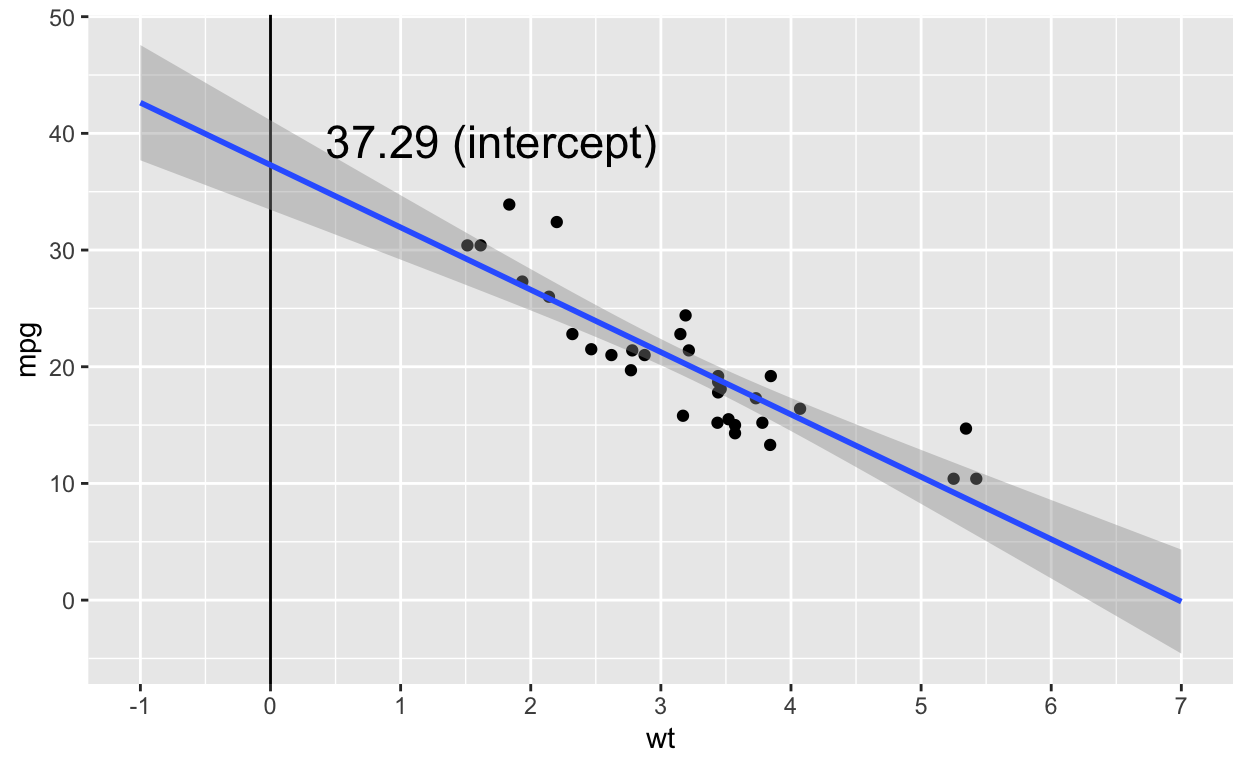## Linear regression with one categorical predictor (two levels)

``````
head(dt1)  # check data (vs is a binary variable with just 0 and 1)``````
``````
mpg cyl disp  hp drat    wt  qsec vs am gear carb
1: 21.0   6  160 110 3.90 2.620 16.46  0  1    4    4
2: 21.0   6  160 110 3.90 2.875 17.02  0  1    4    4
3: 22.8   4  108  93 3.85 2.320 18.61  1  1    4    1
4: 21.4   6  258 110 3.08 3.215 19.44  1  0    3    1
5: 18.7   8  360 175 3.15 3.440 17.02  0  0    3    2
6: 18.1   6  225 105 2.76 3.460 20.22  1  0    3    1``````
``````
dt1[, vs_factor := as.factor(vs)]  # turn vs into a factor
model_categorical_predictor <- lm(mpg ~ vs_factor, dt1)
# summary(model_categorical_predictor)
coef(model_categorical_predictor)``````
``````
(Intercept)  vs_factor1
16.616667    7.940476 ``````

When the categorical predictor has only two levels (coded 0 and 1), we can use the numeric variable as the predictor. We’ll get the same results as above.

``````
coef(lm(mpg ~ vs_factor, dt1))  # factor predictor``````
``````
(Intercept)  vs_factor1
16.616667    7.940476 ``````
``````
coef(lm(mpg ~ vs, dt1))  # numeric predictor``````
``````
(Intercept)          vs
16.616667    7.940476 ``````
• 7.94: whenever `vs_factor` increases by 1 (unit), `mpg` changes by this amount; here, when `vs = 0` is one categorical level/condition, and vs = 1 is the second categorical level/condition; thus this value refers to the difference in mean values between the two conditions
• 16.62: whenever `vs_factor` is 0, `mpg` is this value (i.e., intercept: the value of y when x = 0); thus, the intercept is the mean of the values when `vs = 0`.

To show you the interpretation of the coefficients is indeed correct, let’s manually compute the mean of the two conditions (`vs = 0`, `vs = 1`) and compute their difference.

``````
# compute mean mpg for each vs condition
vs_condition_means <- dt1[, .(mpg_group_mean = mean(mpg)), keyby = vs]
vs_condition_means  ``````
``````
vs mpg_group_mean
1:  0       16.61667
2:  1       24.55714``````

The mean `mpg` value for the group `vs = 0` is the same as the intercept value from the regression above (16.62).

``````
# compute difference in mpg value between vs conditions
vs_condition_means\$mpg_group_mean - vs_condition_means\$mpg_group_mean``````
``````
 7.940476``````

The difference in mean `mpg` values between the two `vs` conditions is the same as the slope (beta coefficient) from the regression above (7.94).

``````
ggplot(dt1, aes(vs, mpg)) +
geom_point() +
geom_smooth(method = 'lm', formula = y ~ poly(x, 1), fullrange = TRUE)``````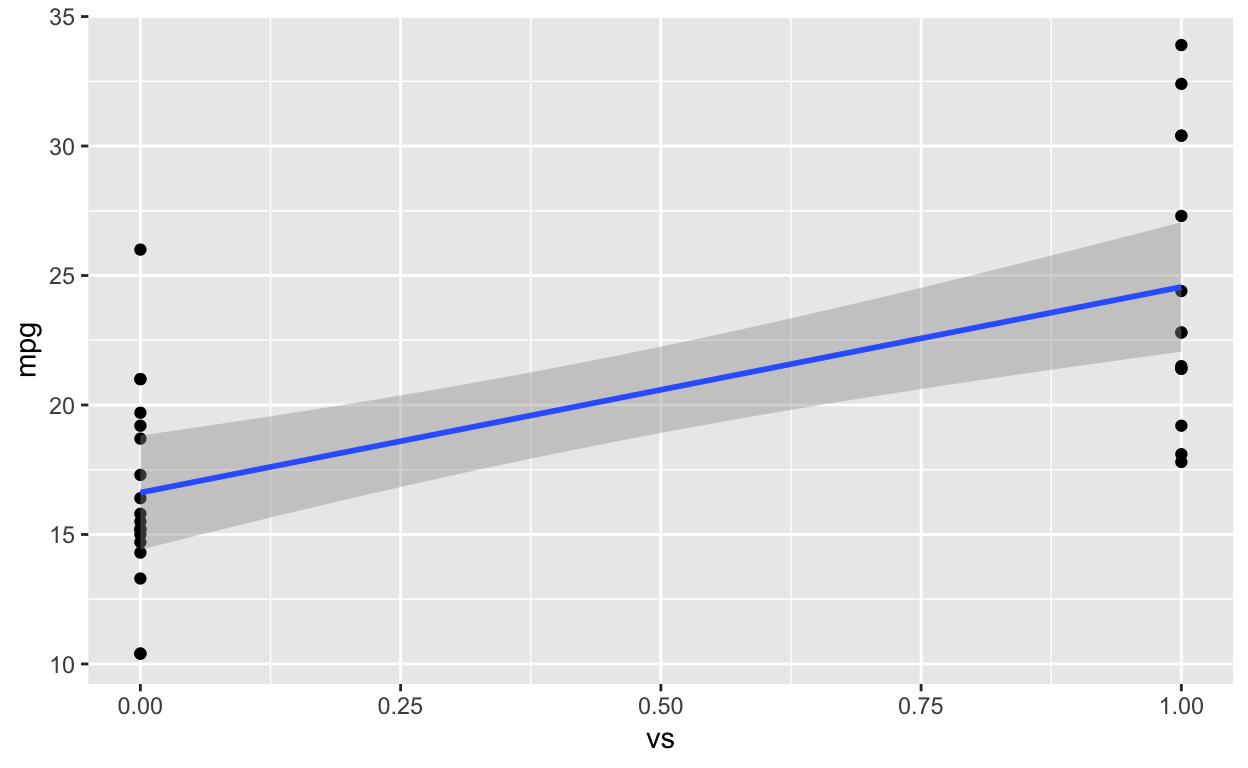## Linear regression with one categorial predictor (three levels)

``````
head(dt1)  # check data (cyl is a categorical predictor with 3 levels)``````
``````
mpg cyl disp  hp drat    wt  qsec vs am gear carb vs_factor
1: 21.0   6  160 110 3.90 2.620 16.46  0  1    4    4         0
2: 21.0   6  160 110 3.90 2.875 17.02  0  1    4    4         0
3: 22.8   4  108  93 3.85 2.320 18.61  1  1    4    1         1
4: 21.4   6  258 110 3.08 3.215 19.44  1  0    3    1         1
5: 18.7   8  360 175 3.15 3.440 17.02  0  0    3    2         0
6: 18.1   6  225 105 2.76 3.460 20.22  1  0    3    1         1``````
``````
dt1[, cyl_factor := as.factor(cyl)]  # turn cyl into a factor
model_categorical_predictor_3 <- lm(mpg ~ cyl_factor, dt1)
# summary(model_categorical_predictor_3)
coef(model_categorical_predictor_3)``````
``````
(Intercept) cyl_factor6 cyl_factor8
26.663636   -6.920779  -11.563636 ``````

When the categorical predictor has three or more levels, we can’t use the numeric variable as the predictor because the coefficients will be different.

``````
coef(lm(mpg ~ cyl, dt1))  # numeric predictor``````
``````
(Intercept)         cyl
37.88458    -2.87579 ``````
``````
coef(lm(mpg ~ cyl_factor, dt1))  # factor predictor``````
``````
(Intercept) cyl_factor6 cyl_factor8
26.663636   -6.920779  -11.563636 ``````

Interpreting the coefficients in the model with the categorical predictor

• When we convert variables to factors or characters, `R` automatically represents the “smallest” condition (1 is smaller than 9; “a” is smaller than “b”) as the intercept. In other words, this condition is treated assigned the value 0 and all other conditions are assigned 1. That is, `R` by default uses “dummy coding”.

• 26.66: when `cyl_factor` is 4 (or the “smallest” `cyl_factor` value in the dataset), `mpg` is this value (i.e., intercept); thus, the intercept is the mean of the values when `cyl_factor = 4`.

• -6.92: difference in mean `mpg` values between the conditions `cyl_factor = 4` and `cyl_factor = 6`

• -11.56: difference in mean `mpg` values between the conditions `cyl_factor = 4` and `cyl_factor = 8`

To show you the interpretation of the coefficients is indeed correct, let’s manually compute the mean of the three conditions (`cyl_factor` is 4, 6, 8) and compute their differences.

``````
# compute mean mpg for each vs condition
cyl_condition_means <- dt1[, .(mpg_group_mean = mean(mpg)), keyby = cyl_factor]
cyl_condition_means  ``````
``````
cyl_factor mpg_group_mean
1:          4       26.66364
2:          6       19.74286
3:          8       15.10000``````

The mean `mpg` value for the group `cyl_factor = 4` is the same as the intercept value from the regression above.

``````
# compute difference in mpg value between cyl = 6 and cyl = 4
cyl_condition_means\$mpg_group_mean - cyl_condition_means\$mpg_group_mean``````
``````
 -6.920779``````
``````
coef(model_categorical_predictor_3)  # beta coefficient``````
``````
cyl_factor6
-6.920779 ``````
``````
# compute difference in mpg value between cyl = 8 and cyl = 4
cyl_condition_means\$mpg_group_mean - cyl_condition_means\$mpg_group_mean``````
``````
 -11.56364``````
``````
coef(model_categorical_predictor_3)  # beta coefficient``````
``````
cyl_factor8
-11.56364 ``````
``````
ggplot(dt1, aes(cyl, mpg)) +
geom_point() +
geom_smooth(method = 'lm', formula = y ~ poly(x, 1), fullrange = TRUE)``````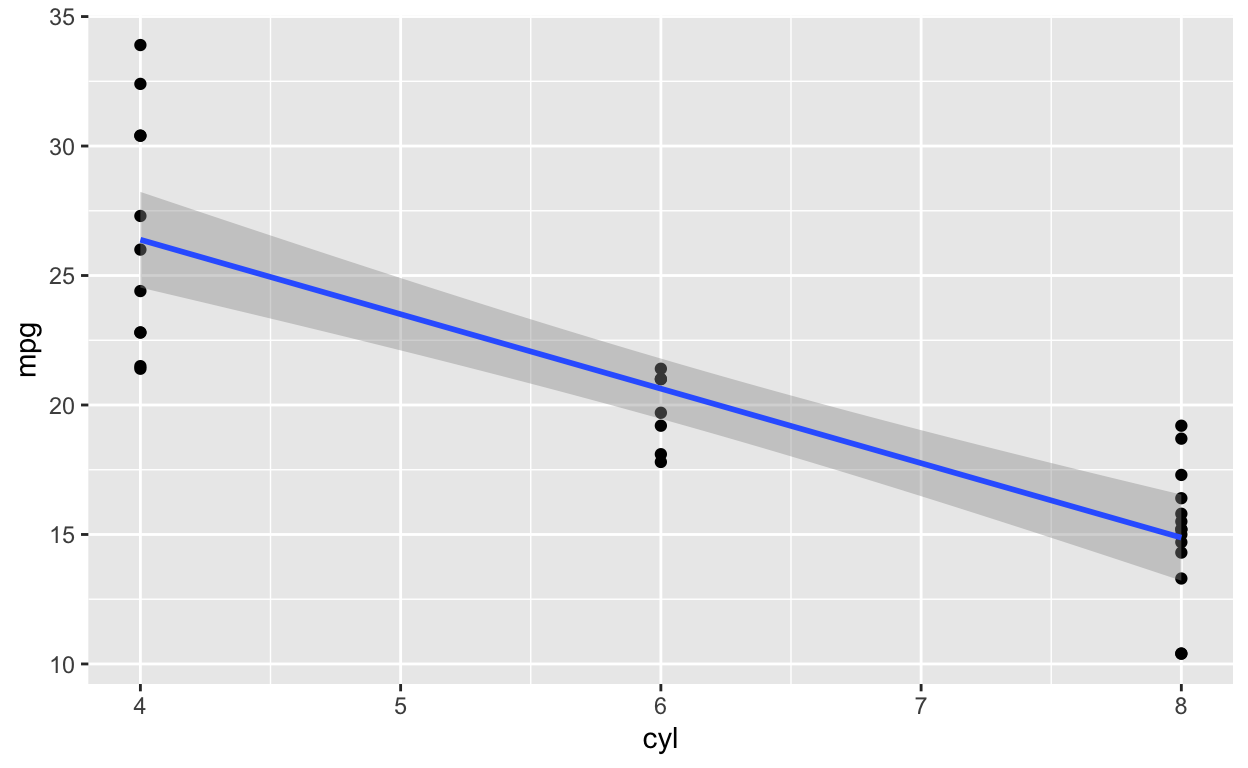When fitting the regression model, `R` uses dummy coding by default. Hence, the condition `cyl = 4` is actually assigned 0 (and thus is the intercept).

## Linear regression with continuous predictor, categorical predictor (two levels), and their interaction

Let’s fit a regression model that includes an interaction term.

``````
model_interaction1 <- lm(mpg ~ disp * vs_factor, data = dt1)
coef(model_interaction1)``````
``````
(Intercept)            disp      vs_factor1 disp:vs_factor1
25.63755459     -0.02936965      8.39770888     -0.04218648 ``````

How do we interpret the interaction coefficient?

For every 1 unit increase in `vs_factor` (coded 0 and 1), the coefficient of `disp` changes by -0.042. READ THAT SENTENCE AGAIN TO SLOWLY DIGEST IT! It’s the change in the COEFFICIENT of `disp` when `vs_factor` increases by 1 (unit).

Let’s fit separate models for the two `vs_factor` conditions to verify the statement/interpretation above.

Fit linear models (`mpg ~ disp`) separately for `vs_factor = 0` and `vs_factor = 1`.

``````
model_mpg_disp_vs0 <- lm(mpg ~ disp, data = dt1[vs_factor == 0])  # blue line in figure below
model_mpg_disp_vs1 <- lm(mpg ~ disp, data = dt1[vs_factor == 1])  # orange line in figure below``````

Check the coefficeints of `disp` for these two models

``````
coef(model_mpg_disp_vs0)``````
``````
(Intercept)        disp
25.63755459 -0.02936965 ``````
``````
coef(model_mpg_disp_vs1)``````
``````
(Intercept)        disp
34.03526346 -0.07155613 ``````

Here’s a reminder (again) of how to interpret the `disp * vs_factor` interaction coefficient in the interaction model (`mpg ~ disp * vs_factor`): For every 1 unit increase in `vs_factor` (coded 0 and 1), the coefficient of `disp` changes by -0.042. Or the change in the COEFFICIENT of `disp` when `vs_factor` increases by 1 (unit).

Let’s compute the difference of the `disp` coefficients in the two models above (where `vs` is 0 and 1).

``````
coef(model_mpg_disp_vs1)['disp'] - coef(model_mpg_disp_vs0)['disp']``````
``````
disp
-0.04218648 ``````

The difference in the `disp` coefficients (-0.042) in the two models (where `vs_factor` is 1 or 0) is identical to the interaction coefficient (`disp * factor`: -0.042) in the `model_interaction1` model.

In other words, the interaction coefficient is the difference between the values of the two slopes (i.e., coefficients) (see figure below).

• `mpg ~ disp` when `vs_factor = 0`: `disp` coefficient is -0.029
• `mpg ~ disp` when `vs_factor = 1`: `disp` coefficient is -0.072
• The slope (i.e., coefficient) of `disp` in the `mpg ~ disp` model is more negative (by `disp * factor`: -0.042) when `vs_factor = 1` than when `vs_factor = 0`.
``````
interact_plot(model_interaction1, pred = disp, modx = vs_factor)``````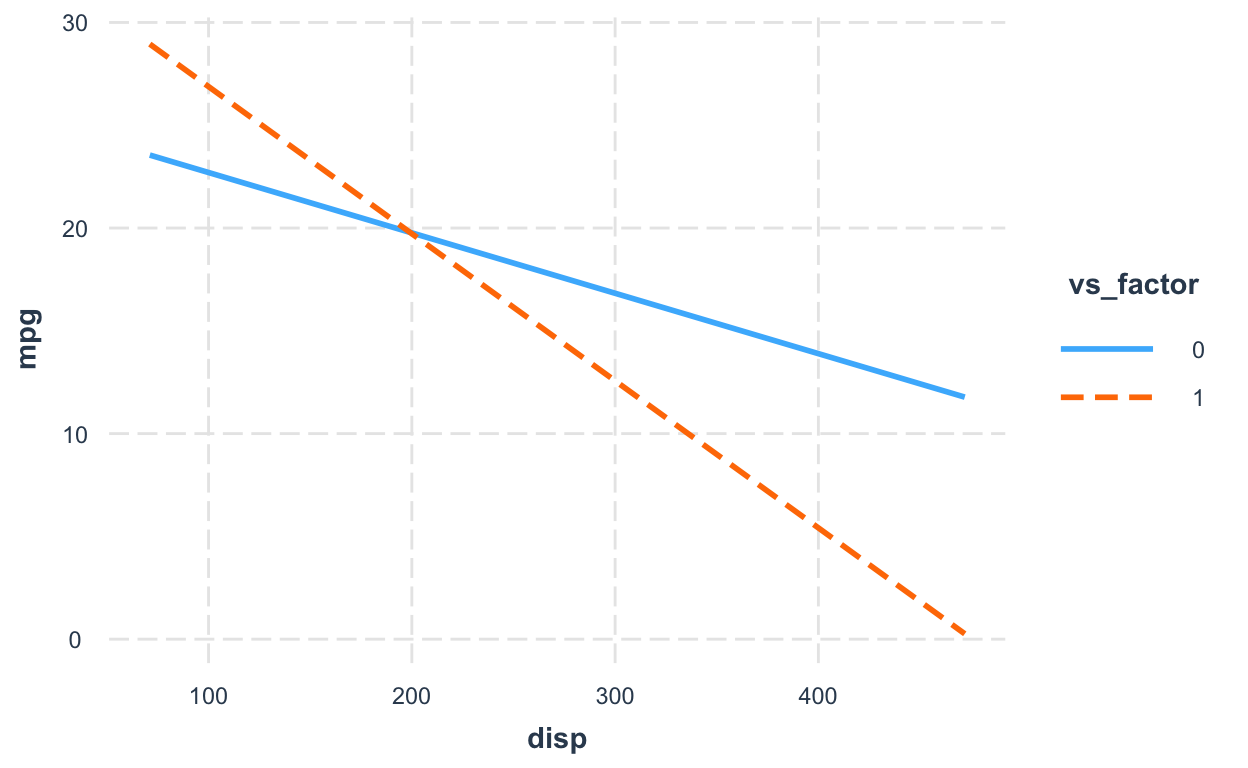## Linear regression with two continuous predictors and their interaction

You can interpret the interaction coefficients in all models (continuous or categorical variables) the same way.

``````
model_interaction2 <- lm(mpg ~ disp * wt, data = dt1)  # all continuous predictors
coef(model_interaction2)``````
``````
(Intercept)        disp          wt     disp:wt
44.08199770 -0.05635816 -6.49567966  0.01170542 ``````
• `disp:wt` = 0.012: the change in the coefficient of `disp` when `wt` increases by 1 unit (or the reverse is also fine: the change in the coefficient of `wt` when `disp` increases by 1 unit)

When all predictors are continuous variables, the convention is to plot the effect of one regressor at different levels (+/- 1 SD and mean value) of the other regressor.

``````
interact_plot(model_interaction2, pred = wt, modx = disp)``````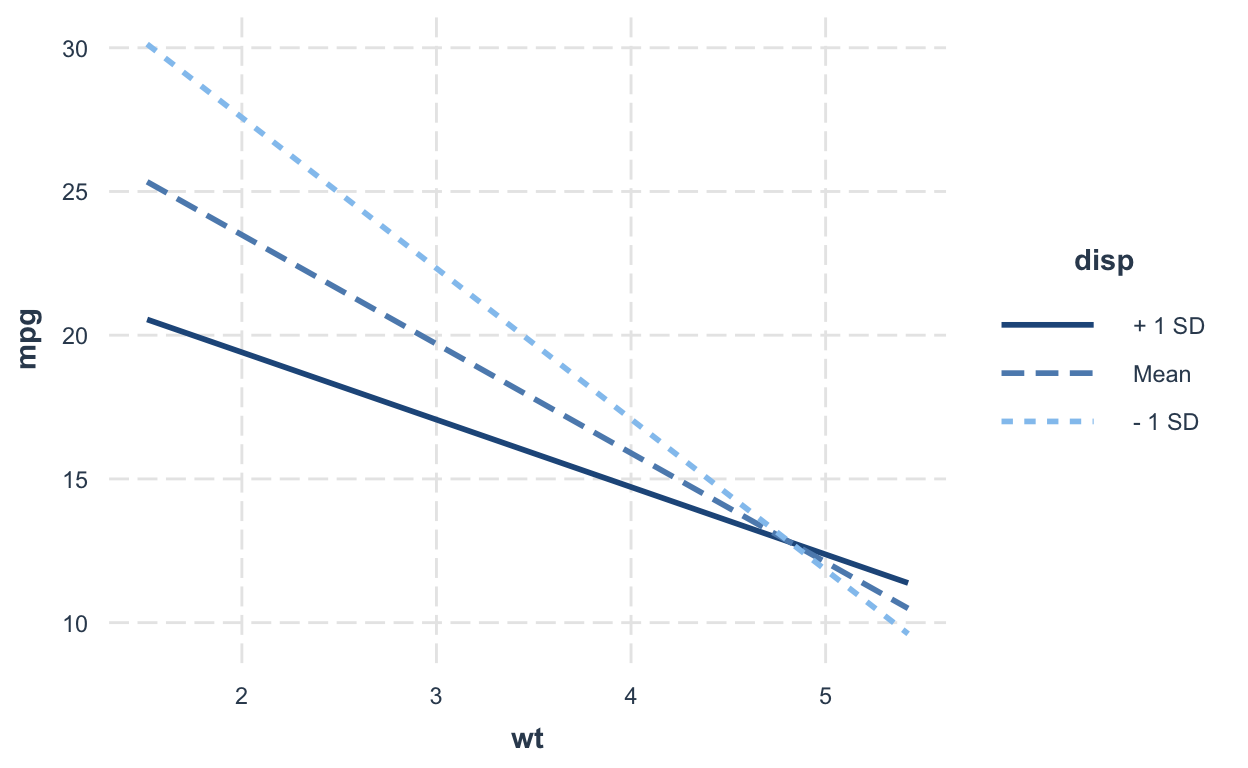``````
interact_plot(model_interaction2, pred = disp, modx = wt)``````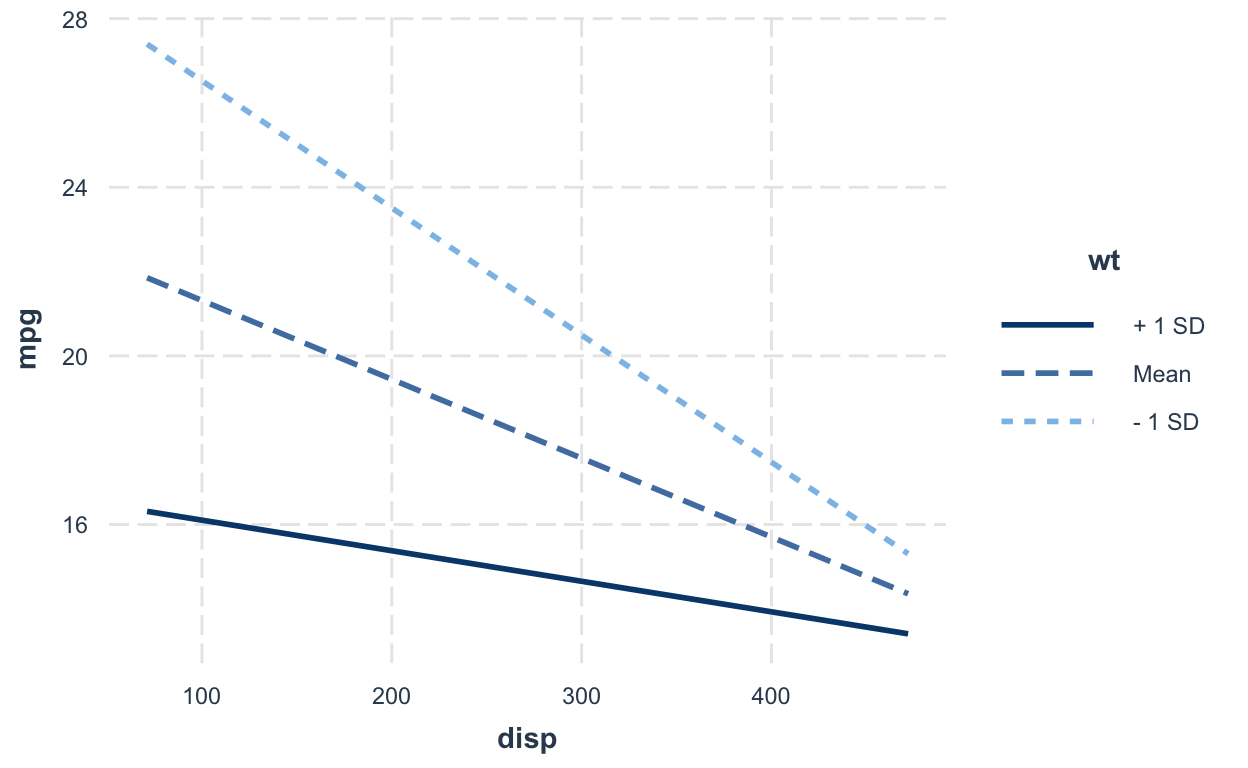## Linear regression with three- or four-way interactions

No matter how complicated your interaction terms are (3 or 4 or 10-way interactions), you interpret the coefficients the same way!

``````
model_interaction4 <- lm(mpg ~ disp * wt * qsec * drat, data = dt1)  # all continuous predictors
coef(model_interaction4)["disp:wt:qsec:drat"]  # the 4-way interaction``````
``````
disp:wt:qsec:drat
0.0301177 ``````

There are many ways to interpret the coefficient `disp:wt:qsec:drat` = 0.03:

• when `disp` increases by 1, the `wt:qsec:drat` coefficient (slope) changes by 0.03
• when `wt` increases by 1, the `disp:qsec:drat` coefficient (slope) changes by 0.03
• when `qsec` increases by 1, the `disp:wt:drat` coefficient (slope) changes by 0.03
• when `drat` increases by 1, the `disp:wt:qsec` coefficient (slope) changes by 0.03

You can also interpret the three- or two-way interactions in the same model in the same way. You get it…

## Support my work

### Corrections

If you see mistakes or want to suggest changes, please create an issue on the source repository.

### Reuse

Text and figures are licensed under Creative Commons Attribution CC BY 4.0. Source code is available at https://github.com/hauselin/rtutorialsite, unless otherwise noted. The figures that have been reused from other sources don't fall under this license and can be recognized by a note in their caption: "Figure from ...".

### Citation

`Lin (2019, July 6). Data science: Interpreting regression coefficients (including interaction coefficients). Retrieved from https://hausetutorials.netlify.com/posts/2019-07-06-interpreting-interaction-regression-coefficients/`
```@misc{lin2019interpreting,